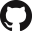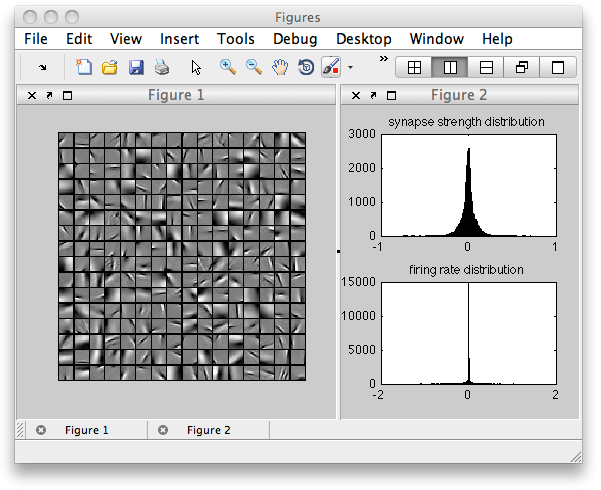[UPDATE: February 2014] The files and instructions are now hosted on GitHub.This minimal set of MATLAB functions will set up a simple neural network to learn receptive fields. These receptive fields minimise an energy function which involves a) image patch reconstruction error, b) sum of absolute firing rates, c) sum of absolute synaptic strengths. We haven’t done this explicitly, but this can be interpreted within a Bayesian framework where the constraints on synapses and firing rates represent some prior distribution over parameters.

Included is a sample of nearly 50,000 16×16 pixel image patches which were randomly sampled from the van Hateren image database (which doesn’t seem to be available online any more, but are possibly mirrored elsewhere). You can of course make your own set of image samples, and the code will work with whatever size image patches you give it. Although it assumes the image patches are square.Instructions:

• Download the MATLAB code and the image patch dataset [here from GitHub], unzip, and place in a folder.
• Set the MATLAB path to that folder
• Run the following code in the command window, adjusting the parameters as you like.
% initialise the network
synapse_cost        = 0.05; % try around 0.03 for starters
firing_rate_cost    = 0;    % try around 0.2 for starters
num_neurons         = 64;
[net, IMAGES]       = ini(num_neurons,synapse_cost,firing_rate_cost);
• Type the following into the command line and it will start iterations of the learning algorithm. If you want you can create your own mfile to do more clever things such as stepwise decrease the learning rate over time.
for n=1:50000, [net]=learn(net,IMAGES); end

Notes:

• I would recommend starting with costs on EITHER synapses OR firing rates. These parameters amount to a dimensionless constraint, so a value of zero means unconstrained.
• The image patches are 16×16, so there are a total of 256 input pixels. So if you want to examine under-complete codes, then set num_neurons to any value under 256. Likewise for over-complete codes set it to any value above 256.
• From our (me and Roland Baddeley’s) research, if you want something that looks like biological reality, then synaptic costs seem to be relevant in the retinal (under-complete) and firing rates seem to be relevant in V1 (over-complete) codes.
• This code trains the network using gradient descent, which is not the fastest method, however the code is as minimal (and hopefully understandable) as possible. You will have to run for a lot of iterations before you approach the global minimum, and you might have to manually decrease net.lr when the receptive fields look like they have begun to converge. If the receptive fields are changing too much or are unstable, then also decreasenet.lr.

If you use this code, please cite both these papers. Thanks!